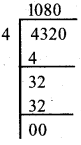KSEEB Solutions for Class 5 Maths Chapter 2 Division

Students can Download Maths Chapter 2 Division Questions and Answers, Summary, Notes Pdf, KSEEB Solutions for Class 5 Maths helps you to revise the complete Karnataka State Board Syllabus and score more marks in your examinations.

Karnataka State Syllabus Class 5 Maths Chapter 2 Division

KSEEB Class 5 Maths Division Revision Exercise

I. Encircle the objects in as shown in the example and write answer in given box: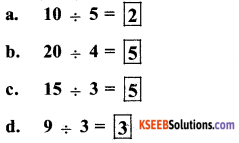II. Divide the following using repeated substruction method:

a. 12 ÷ 4
12 – 4 = 8
8 – 4 = 4
4 – 4 = 0
Subtract is done 3 times
$$\frac{12}{4}$$ = 3b. 25 ÷ 5
25 – 5 = 20
20 – 5 = 15
15 – 5 = 10
10 – 5 = 5
5 – 5 = 0
Subtract is done 5 times
25 ÷ = 5

c. 42 ÷ 7
42 – 7 = 35
35 – 7 =28
28 – 7 = 21
21 – 7 = 14
14 – 7 = 7
7 – 7 = 0
Subtract is done 6 times

d. 30 ÷ 10
30 – 10 = 20
20 – 10 = 10
10 – 10 = 0
Subtract is done 3 times
30 ÷ 10 = 3

e. 75 ÷ 15
75 – 10 = 60
60 – 15 = 45
45 – 15 = 30
30 – 15 = 15
15 – 15 = 0
Subtract is done 5 times
5 ÷ 15 = 5

KSEEB Class 5 Maths Division Ex 2.1

I. Find the quotient and the remainder:

1. 48 ÷ 6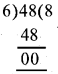Quotient = 8
Remainder =0

2. 36 ÷ 3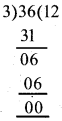Dividend = (divisor × quotient) + Remainder
36 = (3 × 12) +0
36 = 36 + 0
36 = 36
Quotient = 12
Remainder =0

3. 55 ÷ 4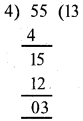Dividend = (divisor × quotient) + Remainder
55 = (4 × 13) + 3
55 = 52 + 3
55 = 55
quotient = 13
Remainder = 3

4. 72 ÷ 7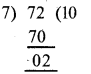Dividend = (divisor × quotient) + Remainder
72 = (7 × 10) + 2
72 = 70 + 2
72 = 72
quotient = 10
Remainder = 02

II. Find the quotient and the remainder:

1. 232 ÷ 4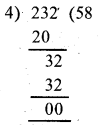Dividend = (divisor × quotient) + Remainder
232 = (4 × 58) + 0
232 = 232 + 0
232 = 232
quotient = 58
Remainder = 32. 474 ÷ 6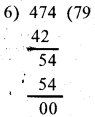Dividend = (divisor × quotient) + Remainder
474 = (6 × 79) + 0
474 = 474 + 0
474 = 474
quotient = 79 3
Remainder =0

3. 255 ÷ 11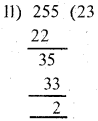Dividend = (divisor × quotient) + Remainder
255 = (11 × 23) + 2
255 = 253 + 2
255 = 255
quotient = 23
Remainder = 2

4. 527 ÷ 12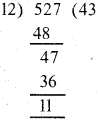Dividend = (divisor × quotient) + Remainder
527 = (12 × 43) + 11
527 = 516 + 11
527 = 527
quotient = 43
Remainder = 11

III. Find the quotient and the remainder:

1. 1,653 ÷ 8Dividend = (divisor × quotient) + Remainder
1653 = (8 × 206) + 5
1653 = 1648 + 5
1653 = 1653
quotient = 206
Remainder = 5

2. 1,325 ÷ 2Dividend = (divisor × quotient) + Remainder
1325 = (2 × 662) + 1
1325 = 1324 + 1
1325 = 1325
quotient = 662
Remainder = 1

3. 1,435 ÷ 15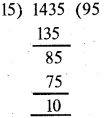Dividend = (divisor × quotient) + Remainder
1435 = (15 × 95) + 10
1435 = 1425+10
1435 = 1435
quotient = 95
Remainder =104. 2,647 ÷ 13Dividend = (divisor × quotient) + Remainder
2647 = (13 × 203) + 8
2647 = 2639 + 8
2647 = 2647
quotient = 203
Remainder = 8

IV. Find the quotient and the remainder:

1. 24,658 ÷ 2Dividend = (divisor × quotient) + Remainder
24658 = (2 × 12329) + 0
24658 = 24658 + 0
24658 = 24658
quotient = 12329
Remainder = 0

2. 14,005 ÷ 7Dividend = (divisor × quotient) + Remainder
14005 = (7 × 2000) + 5
14005 = 14000 + 5
14005 = 14005
quotient = 2000
Remainder = 5

3. 32,745 ÷ 10Dividend = (divisor × quotient) + Remainder
32745 = (10 × 3274) + 5
32745 = 32740 + 5
32745 = 32745
quotient = 3274
Remainder =54. 12,056 ÷ 12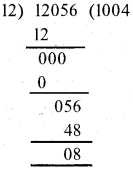Dividend = (divisor × quotient) + Remainder
12056 = (12 × 1004) +8
12056 = 12048 + 8
12056 = 12056
quotient = 1004
Remainder = 8

V. Solve the following problems:

Question 1.
A tailor has 18 metres of cloth. He stitch 9 shirts from this cloth. Find the length of cloth required to stitch one shirt.
A tailor has 18 meter of cloth
Tailor has stitch 9 shirts
The length of cloth required to stitch one shirt = $$\frac{18}{9}$$ = 2 meter

Question 2.
A bike can cover 240 km with 5 litres of petrol. Find how many kilometres does it cover with 1 litre of petrol.
A bike can cover 240 Km with 5 litres of petrol
In one litre of petrol, bike covers the distance = $$\frac{240}{5}$$ = 48 kmQuestion 3.
25,004 bags of cement are loaded equally into 14 railway wagons. Find the number of bags in each wagon.
25,004 bags of cement are loaded equally into 14 railway wagons
The number of bags
= $$\frac{25004}{14}$$ = 1,786Question 4.
Mr. Sridhar purchased 11 toys of same price for Rs. 946 from a toy shop. Find the cost of each toy.
The cost of a toy =?
Mr. Sridhar purchase 11 toys of same price = Rs 946
The cost of each toy
= $$\frac{946}{11}$$ = 86 Rs.

Question 5.
The annual income of a person is Rs.90,912. Find his monthly income.
The Annual income of a peusen Rs 90,912
Monthly income = $$\frac{90912}{12}$$ = 7,576 RsQuestion 6.
A car manufacturing company manufactures 14,820 cars in 13 months. Find the number of cars manufactured in one month.
A car manufacturing company manufactures 14820 cars in 13 months. For one month, the number of cars = $$\frac{14820}{13}$$ = 1,140KSEEB Class 5 Maths Division Ex 2.2

I. Solve

Question 1.
An orange garden has 82 orange plants. A farmer plucks 60 oranges from each plant and packs 12 oranges in each box. Calculate the number of boxes required to pack all oranges.
An orange garden has 82 oranges . A farmer plunks 60 oranges from each plant
The number of boxes = ?
The number of oranges in each box = 12
The number of oranges in an orange garden = 410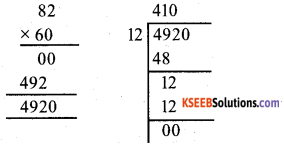Question 2.
15 school children hire a cab for a day’s excursion at Rs. 9 per km. If they travel a distance of 325 km, find the amount to be shared by each one of them.
A cab of charges Rs 9 Km
The childrens travel a distance 325Km
= 325 × 9
= 2925 Km
The amount shared by each student
= 2925 ÷ 15 = Rs 195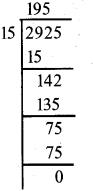Question 3.
Anita has a cow which yields 8 litres of milk in a day. The selling price of one litre of milk is Rs. 18. She wants to divide the amount earned in one month (30days) among her 4 sons. What amount does each one get?